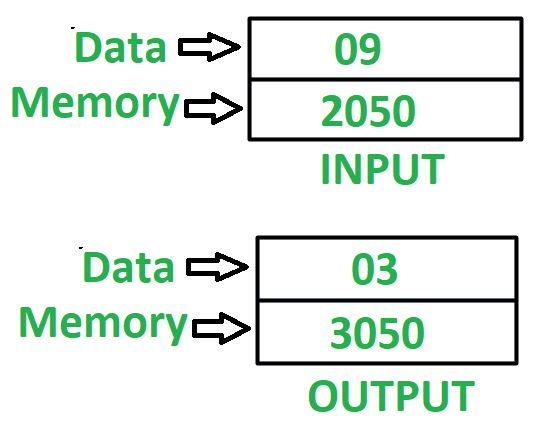Open In App

# 8085 program to find square root of a number

Problem – Write an assembly language program in 8085 microprocessor to find square root of a number. Example –Assumptions – Number, whose square root we need to find is stored at memory location 2050 and store the final result in memory location 3050. Algorithm –

1. Assign 01 to register D and E
2. Load the value, stored at memory location 2050 in accumulator A
3. Subtract value stored at accumulator A from register D
4. Check if accumulator holds 0, if true then jump to step 8
5. Increment value of register D by 2
6. Increment value of register E by 1
8. Move value stored at register E in A
9. Store the value of A in memory location 3050

Program –

Explanation – Registers used A, D, E:

1. MVI D, 01 – initialize register D with 01
2. MVI E, 01 – initialize register E with 01
3. LDA 2050 – loads the content of memory location 2050 in accumulator A
4. SUB D – subtract value of D from A
5. JZ 2011 – make jump to memory location 2011 if zero flag is set
6. INR D – increments value of register D by 1. Since it is used two times, therefore value of D is incremented by 2
7. INR E – increments value of register E by 1
9. MOV A, E – moves the value of register E in accumulator A
10. STA 3050 – stores value of A in 3050
11. HLT – stops executing the program and halts any further execution

### Advantages of finding the square root:

• It is an important mathematical operation used in various fields, such as engineering, physics, and finance.

• It can be used to find the distance between two points in a coordinate plane, the length of a side of a square, or the velocity of an object.

• It is a fundamental operation used in more advanced mathematical operations, such as calculus.

### Disadvantages of finding the square root:

• It can be a time-consuming and complex operation, especially for larger numbers.

• Some methods for finding the square root may not always provide an exact answer and may require rounding or approximations.

• The accuracy of the result may depend on the chosen method and the number of iterations used.